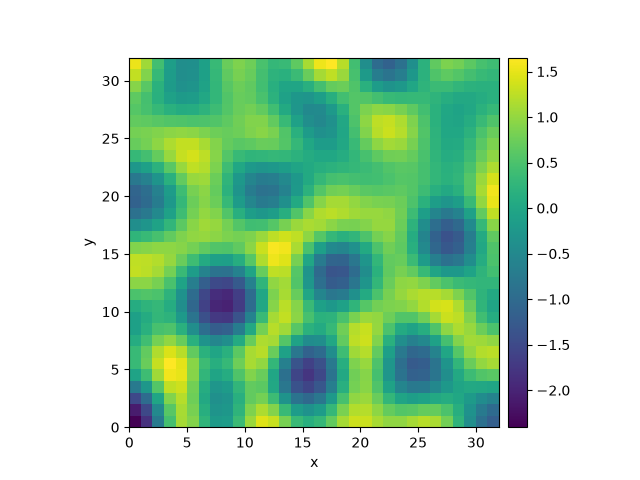# 2.23. Kuramoto-Sivashinsky - Compiled methods

This example implements a scalar PDE using a custom class with a numba-compiled method for accelerated calculations. We here consider the Kuramoto–Sivashinsky equation, which for instance describes the dynamics of flame fronts:

$\partial_t u = -\frac12 |\nabla u|^2 - \nabla^2 u - \nabla^4 u$0%|          | 0/10.0 [00:00<?, ?it/s]
Initializing:   0%|          | 0/10.0 [00:00<?, ?it/s]/home/docs/checkouts/readthedocs.org/user_builds/py-pde/checkouts/latest/examples/pde_custom_numba.py:39: NumbaDeprecationWarning: The 'nopython' keyword argument was not supplied to the 'numba.jit' decorator. The implicit default value for this argument is currently False, but it will be changed to True in Numba 0.59.0. See https://numba.readthedocs.io/en/stable/reference/deprecation.html#deprecation-of-object-mode-fall-back-behaviour-when-using-jit for details.
def pde_rhs(data, t):

0%|          | 0/10.0 [00:11<?, ?it/s]
0%|          | 0.01/10.0 [00:12<3:29:38, 1259.09s/it]
0%|          | 0.02/10.0 [00:12<1:44:43, 629.57s/it]
2%|▏         | 0.17/10.0 [00:12<12:08, 74.07s/it]
70%|██████▉   | 6.99/10.0 [00:12<00:05,  1.80s/it]
70%|██████▉   | 6.99/10.0 [00:12<00:05,  1.80s/it]
100%|██████████| 10.0/10.0 [00:12<00:00,  1.26s/it]
100%|██████████| 10.0/10.0 [00:12<00:00,  1.26s/it]


import numba as nb

from pde import PDEBase, ScalarField, UnitGrid

class KuramotoSivashinskyPDE(PDEBase):
"""Implementation of the normalized Kuramoto–Sivashinsky equation"""

def __init__(self, bc="auto_periodic_neumann"):
super().__init__()
self.bc = bc

def evolution_rate(self, state, t=0):
"""implement the python version of the evolution equation"""
state_lap = state.laplace(bc=self.bc)
state_lap2 = state_lap.laplace(bc=self.bc)
return -state_grad_sq / 2 - state_lap - state_lap2

def _make_pde_rhs_numba(self, state):
"""nunmba-compiled implementation of the PDE"""
laplace = state.grid.make_operator("laplace", bc=self.bc)

@nb.jit
def pde_rhs(data, t):
return -0.5 * gradient_squared(data) - laplace(data + laplace(data))

return pde_rhs

grid = UnitGrid([32, 32])  # generate grid
state = ScalarField.random_uniform(grid)  # generate initial condition

eq = KuramotoSivashinskyPDE()  # define the pde
result = eq.solve(state, t_range=10, dt=0.01)
result.plot()


Total running time of the script: (0 minutes 12.795 seconds)